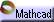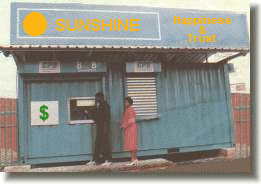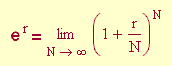Example 3: Discrete Accumulation Click here to open an associated Mathcad worksheet:Imagine that you wish to invest \$100 in a local bank account. The interest rates at the local banks are controlled by market conditions, and all of the banks in your area are offering the same annual rate of 4% on savings accounts. This means that, if the interest were computed after one year, your investment would be worth:

100 + (0.04)(100) = \$104.00

for a net increase in principle of exactly four dollars.

You notice, however, that different banks are offering different numbers of compoundings during the year. With compounding, part of the annual interest on an account is computed before the end of the year – perhaps several times before the end of the year. The increase in principle at each compounding is added to the account immediately, so that it will figure in future compoundings. Compounding results in an effective annual rate (also known as the yield) that is greater than 4%. The more times an account is compounded during the year, the higher the effective rate.

Of the three banks in your area, Corporate Bank offers four compoundings a year (quarterly compounding), Farmer's Credit Union offers twelve (monthly compounding), and Sunshine Happiness & Trust offers ...continuous compounding! Apparently, SH&T will employ an accountant to re-compute your interest every second of every day over the next year. Giddy with the thought of it, you invest your \$100 with SH&T.

Let us, however, make a more considered comparison. At Corporate Bank, your interest would be computed as follows:

 1st Quarter: 100 + (.04/4)(100) = \$101.00 . (Notice that, since the bank will be compounding four times during the year, it uses one-fourth of the annual rate at each compounding.) 2nd Quarter: 101.00 + (.04/4)(101.00) = \$102.01 . 3rd Quarter: 102.01 + (.04/4)(102.01) = \$103.03 . Year's End: 103.03 + (.04/4)(103.03) = \$104.06 .

The quarterly compounding earns you an additional six cents over the same 4% annual rate without compounding. The effective rate is 4.06%, since you effectively add this percentage to your original investment.

At Farmer's Credit Union, your interest would be computed as follows:

 1st Month: 100 + (.04/12)(100) = \$100.33 . 2nd Month: 100.33 + (.04/12)(100.33) = \$100.66 . 3rd Month: 100.66 + (.04/12)(100.66) = \$101.00 . ... see if you can do the intermediate calculations ... Year's End: 103.73 + (.04/12)(103.73) = \$ 104.08 .

The monthly compounding earns you an additional eight cents over the same 4% annual rate without compounding. The effective rate is 4.08%.

How would SH&T compute your interest? There will apparently be a lot of compoundings – let's say  N  of them. Then the interest computations would look like this:

 1st Compounding: 100 + (.04/N)(100) = \$100(1 + .04/N) . 2nd Compounding: 100(1 + .04/N) + (.04/N)(100(1 + .04/N)) = 100(1 + .04/N)(1+ .04/N) = \$100(1 + .04/N)2 . 3rd Compounding: 100(1 + .04/N)2 + (.04/N)(100(1 + .04/N)2) = 100(1 + .04/N)2(1+ .04/N) = \$100(1 + .04/N)3 . ... Year's End (Nth Compounding): \$100(1 + .04/N)N .

Notice that this computation really has nothing to do with  N  being large, and this general year-end formula could just as well have been used for the computations with quarterly and monthly compoundings:

 N = 4: 100(1 + .04/N)N = 100(1 + .04/4)4 = \$104.06 ; N = 12: 100(1 + .04/N)N = 100(1 + .04/12)12 = \$104.08 ;

exactly as before.

But what if the number of compoundings  N  is really large, like at SH&T? Try it. You will see that the year-end formula with  N = 500 , N = 1000 , and  N = 3.15 x 107 (the number of seconds in a year) all give the same result when rounded to the nearest penny: \$104.08 – exactly the same year-end balance as at Farmer's Credit Union. The effective rate apparently approaches a limiting value of  4.08%, even as the number of compoundings grows very large. Continuous compounding, you soberly conclude, is nothing more than a clever marketing scheme.

But what does this have to do with e? Just this:

100·e0.04 = 104.08 !

You can use  e  to quickly compute the limit on the effective rate.

Notice that there was nothing special about the  \$100  and the  4%  that we used in this example. For an initial investment of  P  dollars with an annual interest rate of  r %  compounded  N  times per year, the computation of the balances would proceed in the same way. The result is a year-end accumulation of  P(1 + r/N)N  dollars. As the number of compoundings  N  grows without bound, the number  (1 + r/N)N  approaches the limiting value of  er, and the year-end balance approaches the limiting value of  Per.

We summarize these observations with the following suggestive notation:In particular, for  r = 1 , this formula gives another way to compute the decimal expansion of  e. Try it out for some large values of  N . Do the estimates converge more or less quickly than those produced by the formulas in Example 2?